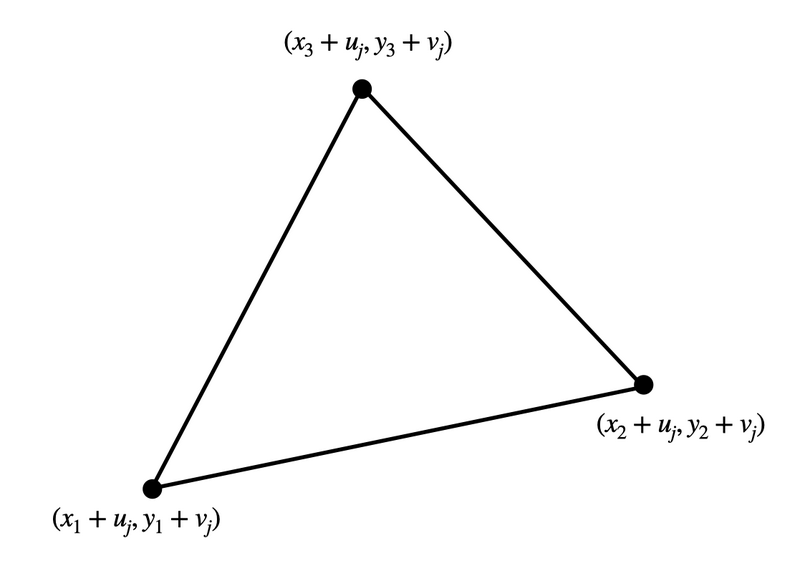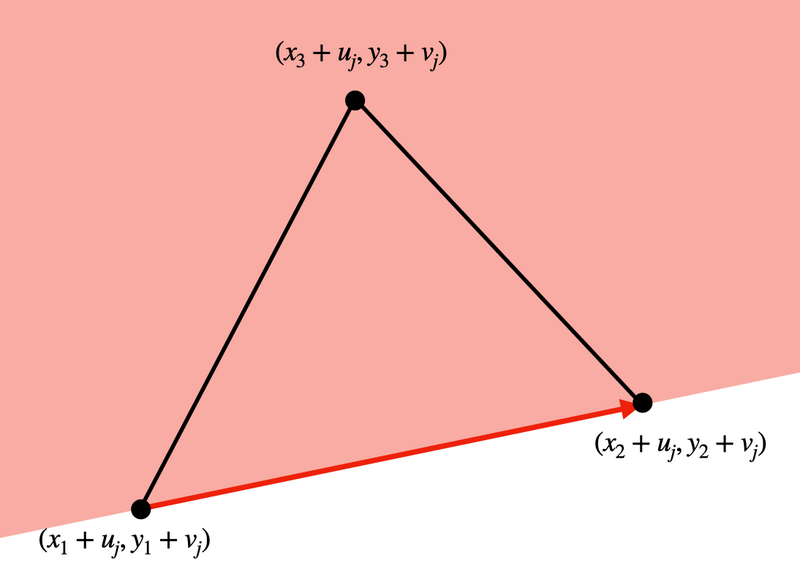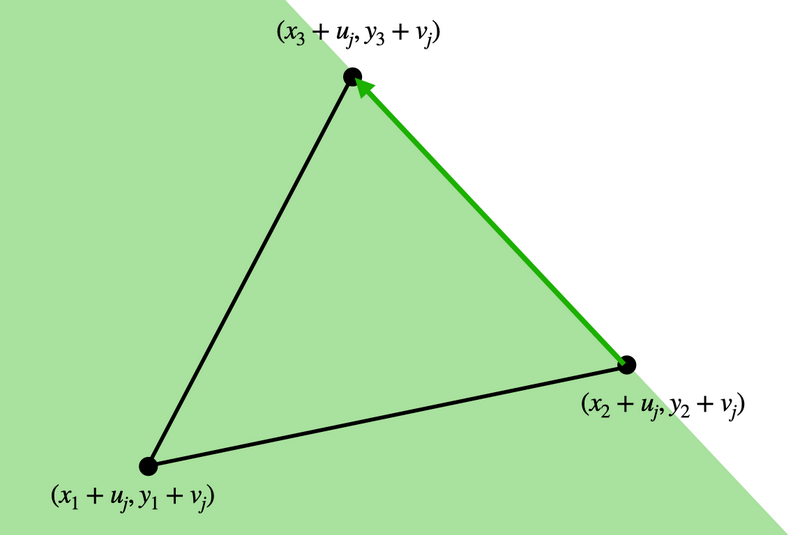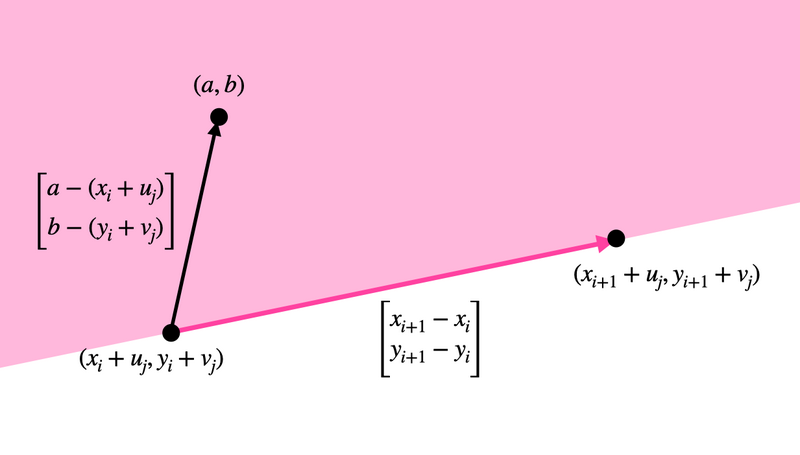Contest Duration: - (local time) (100 minutes) Back to Home

## G - Intersection of Polygons Editorial by en_translator

Let us consider the necessary and sufficient condition that a point $$(a, b)$$ is contained in the polygon $$P_j$$. For example, suppose that $$P_j$$ is a triangle illustrated as follows.Here, a point $$(a, b)$$ is contained in $$P_j$$ if and only if it is contained in all of the following three regions (including the boundaries).In general, a point $$(a, b)$$ is contained in the polygon $$P_j$$ if and only if the following is satisfied for all $$i = 1, 2, \ldots, N$$:

A point $$(a, b)$$ is contained in the left region of the directed segment from the point $$(x_i+u_j, y_i + v_j)$$ to the point $$(x_{i+1}+u_j, y_{i+1} + v_j)$$ (or the boundary).

“A point $$(a, b)$$ is contained in the left region of the directed segment from the point $$(x_i+u_j, y_i + v_j)$$ to the point $$(x_{i+1}+u_j, y_{i+1} + v_j)$$ (or the boundary)” if and only if the vector $$\left[\begin{matrix} a - (x_i+u_j)\\ b - (y_i+v_j)\\ \end{matrix}\right]$$ of the directed segment from the point $$(x_i+u_j, y_i+v_j)$$ to the point $$(x_{i+1}+u_j, y_{i+1}+v_j)$$ and the vector $$\left[\begin{matrix} a - (x_i+u_j)\\ b - (y_i+v_j)\\ \end{matrix}\right]$$ of the directed segment from the point $$(x_i+u_j, y_i+v_j)$$ to the point $$(a, b)$$ (see also the figure below) have the property that

$\left[\begin{matrix} x_{i+1} - x_i\\ y_{i+1} - y_i\\ \end{matrix}\right] \times \left[\begin{matrix} a - (x_i+u_j)\\ b - (y_i+v_j)\\ \end{matrix}\right] \geq 0. \tag{1}$

Here, $$\left[\begin{matrix} p\\ q\\ \end{matrix}\right] \times \left[\begin{matrix} r\\ s\\ \end{matrix}\right]$$ is defined to be the scalar value represented by $$ps - qr$$.We can transform the expression even further as

$\vec{Q_i} := \left[\begin{matrix} x_{i+1} - x_i\\ y_{i+1} - y_i\\ \end{matrix}\right], R_{i, j} := \left[\begin{matrix} x_{i+1} - x_i\\ y_{i+1} - y_i\\ \end{matrix}\right] \times \left[\begin{matrix} x_i+u_j\\ y_i+v_j\\ \end{matrix}\right]$

so (1) is equivalent to

$\vec{Q_i} \times \left[\begin{matrix} a\\ b\\ \end{matrix}\right] \geq R_{i, j}. \tag{2}$

Thus, in order to determine if a point $$(a, b)$$ is contained in $$P_j$$, it is sufficient to determine if (2) holds for all $$i = 1, 2, \ldots, N$$. Therefore, in order to determine if a point $$(a, b)$$ is contained in all of $$P_1, P_2, \ldots, P_M$$, it is sufficient to determine if (2) holds for all pairs of $$i = 1, 2, \ldots, N$$ and $$j = 1, 2, \ldots, M$$.

However, this method requires checking if (2) is satisfied for all $$NM$$ pairs of $$(i, j)$$ for each point $$(a, b)$$, but it is hopeless it can be processed in time for each point $$(a, b)$$, but it is hopeless it can be processed in time.

Here, note that the left hand side of (2) is independent of $$j$$ and only dependent on $$i$$. Thus, instead of checking if (2) holds for all pairs of $$i = 1, 2, \ldots, N$$ and $$j = 1, 2, \ldots, M$$, it is sufficient to check if it holds for all $$i = 1, 2, \ldots, N$$ that

$\vec{Q_i} \times \left[\begin{matrix} a\\ b\\ \end{matrix}\right] \geq \max_{j = 1, 2, \ldots, M} R_{i, j}. \tag{3}$

So, we first spend $$\mathrm{O}(NM)$$ time to precalculate $$\max_{j = 1, 2, \ldots, M} R_{i, j}$$ for all $$i = 1, 2, \ldots, N$$. Then we can determine if (3) is satisfied for a given point $$(a, b)$$ for all $$i = 1, 2, \ldots, N$$ in an $$\mathrm{O}(N)$$ time for each point.

Therefore, the problem has been solved in a total of $$\mathrm{O}(N(M + Q))$$ time.

The following is a sample code in C++.

#include <iostream>
using namespace std;
typedef long long ll;
const ll inf = 1e18;

ll n, m, q;
ll x, y;
ll u, v;
ll Q1, Q2, R;

ll cross(ll p, ll q, ll r, ll s){
return p*s - q*r;
}

int main(void)
{
cin >> n;
for(int i = 1; i <= n; i++) cin >> x[i] >> y[i];
x[n+1] = x, y[n+1] = y;
cin >> m;
for(int i = 1; i <= m; i++) cin >> u[i] >> v[i];

for(int i = 1; i <= n; i++){
Q1[i] = x[i+1]-x[i], Q2[i] = y[i+1]-y[i], R[i] = -inf;
for(int j = 1; j <= m; j++){
R[i] = max(R[i], cross(x[i+1]-x[i], y[i+1]-y[i], x[i]+u[j], y[i]+v[j]));
}
}

cin >> q;
ll a, b;
for(int k = 1; k <= q; k++){
cin >> a >> b;
bool ans = true;
for(int i = 1; i <= n; i++) if(cross(Q1[i], Q2[i], a, b) < R[i]) ans = false;
if(ans) cout << "Yes" << "\n";
else cout << "No" << "\n";
}

return 0;
}


posted:
last update: